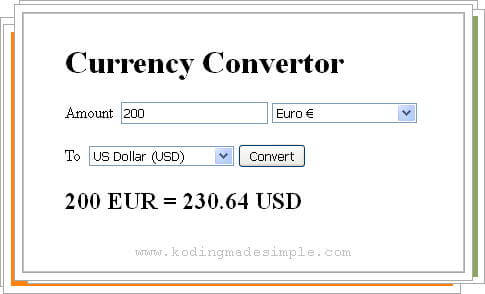# Currency Conversion in PHP using Google Finance API

Hi! In this tutorial, let us see how to convert currency in PHP using Google Finance API. There are lots of currency conversion api's available but I'm going to use Google Finance here. Using the api you can easily convert money to your desired country currency. You have to send out http request to Google finance tagging with three values - amount, from currency and to currency code. And it sends back the response as HTML which you have to parse and extract the converted amount.## PHP Function to Convert Currency:

Following is the currency convertor function I have created. The function takes up 3 params namely 'from_currency', 'to_currency' and 'amt' and makes call to google finance api. It uses `file_get_contents()` function to send http request and receives raw html response. Then it parses the html and returns the converted currency value.

```<?php
function convert_currency(\$from_currency, \$to_currency, \$amt) {
\$from_currency = urlencode(\$from_currency);
\$to_currency = urlencode(\$to_currency);
\$amt = urlencode(\$amt);
\$data = explode('bld>', \$data);
\$data = explode(\$to_currency, \$data);
return round(\$data, 2);
}
?>
```

## Function Usage:

```echo convert_currency(USD, EUR, 100);
```

## How to Convert Currency in PHP?

Now let me show you how to use the above function and implement currency convertor functionality in your application with a demo.

For that we'll need to create two php files, index.php and function.php.

In function.php, we keep the convert_currency() function we have created earlier. And in the index file, add a html form and input fields for amount and two dropdowns to choose from and to currency.

### index.php

```<?php
include_once "function.php";

//check if form is submitted
if (isset(\$_POST["submit"])) {
\$from_curr = \$_POST["from_currency"];
\$to_curr = \$_POST["to_currency"];
\$amount = \$_POST["amount"];
\$result = convert_currency(\$from_curr, \$to_curr, \$amount);
}
?>
<!DOCTYPE html>
<html>
<title>Currency Conversion in PHP</title>
<body>
<h1>Currency Convertor</h1>
<form action="index.php" method="post">
<label for="amount">Amount</label>
<input type="text" name="amount" placeholder="Enter amount" value="<?php if(isset(\$_POST["amount"])){echo \$_POST["amount"];} ?>" required />

<?php
\$options = array(
'GBP' => 'British Pound £',
'EUR' => 'Euro €',
'FRF' => 'French Franc (FRF)',
'DEM' => 'German Mark (DEM)',
'INR' => 'Indian Rupee (INR)',
'USD' => 'US Dollar (USD)'
);
?>
<select name="from_currency">
<?php foreach(\$options as \$key => \$value){ ?>
<option value="<?php echo \$key; ?>" <?php if(isset(\$_POST["from_currency"]) && (\$_POST["from_currency"]==\$key)){echo "selected";} ?>><?php echo \$value; ?></option>
<? } ?>
</select>
<br/><br/>
<label>To</label>
<select name="to_currency">
<?php foreach(\$options as \$key => \$value){ ?>
<option value="<?php echo \$key; ?>" <?php if(isset(\$_POST["to_currency"]) && (\$_POST["to_currency"]==\$key)){echo "selected";} ?>><?php echo \$value; ?></option>
<? } ?>
</select>
<input type="submit" name="submit" value="Convert" />
</form>

<span><h2><?php if (isset(\$result)) { echo \$amount . " " . \$from_curr . " = " . \$result . " " . \$to_curr; } ?></h2></span>
</body>
</html>
```

### function.php

```<?php
function convert_currency(\$from_currency, \$to_currency, \$amt) {
\$from_currency = urlencode(\$from_currency);
\$to_currency = urlencode(\$to_currency);
\$amt = urlencode(\$amt);
\$data = explode('bld>', \$data);
\$data = explode(\$to_currency, \$data);
return round(\$data, 2);
}
?>
```

So user must enter the amount value, from and to currency code and submit the form. And the script sends http request to Google finance, get response, extract and display the converted amount on the page.

Likewise you can implement currency convertor in php application. I hope you find this useful. If you like this tutorial, please don't forget to share it in social media. Meet you in another interesting post.

#### 1 comment:

1.thanks for sharing data. could not execute the following script.
Notice: Undefined offset: 1 in C:\xampp\htdocs\currency.php on line 8 this is the line number 8 script: \$data = explode(\$to_currency, \$data); please help me.

Name

Email *

Message *